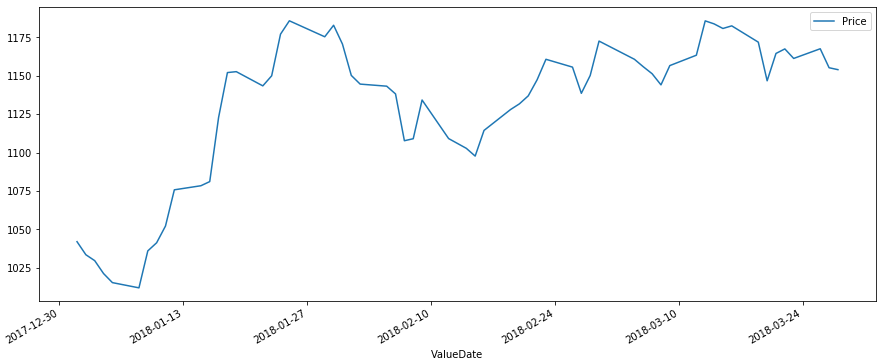# (31)Python时间序列

``````ValueDate    Price
01-01-2018,    1042.05
02-01-2018,    1033.55
03-01-2018,    1029.7
04-01-2018,    1021.3
05-01-2018,    1015.4
...
...
...
...
23-03-2018,    1161.3
26-03-2018,    1167.6
27-03-2018,    1155.25
28-03-2018,    1154
```Python```

## 创建时间序列

``````from datetime import datetime
import pandas as pd
import matplotlib.pyplot as plt

df = pd.DataFrame(data, columns = ['ValueDate', 'Price'])

# Set the Date as Index
df['ValueDate'] = pd.to_datetime(df['ValueDate'])
df.index = df['ValueDate']
del df['ValueDate']

df.plot(figsize=(15, 6))
plt.show()
```Python```